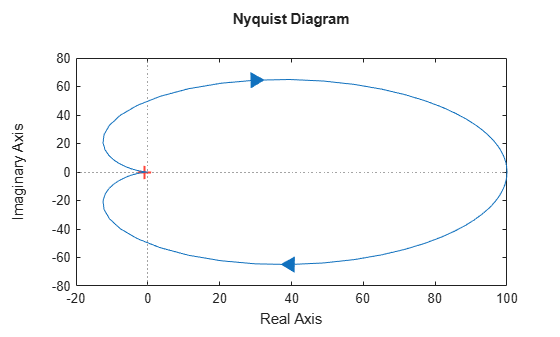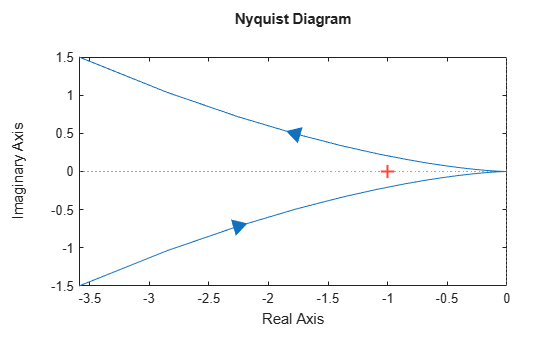# nyquistplot

Nyquist plot with additional plot customization options

## Syntax

```h = nyquistplot(sys) nyquistplot(sys,{wmin,wmax}) nyquistplot(sys,w) nyquistplot(sys1,sys2,...,w) nyquistplot(AX,...) nyquistplot(..., plotoptions) ```

## Description

`h = nyquistplot(sys)` draws the Nyquist plot of the dynamic system model `sys`. It also returns the plot handle `h`. You can use this handle to customize the plot with the `getoptions` and `setoptions` commands. Type

```help nyquistoptions ```

for a list of available plot options.

The frequency range and number of points are chosen automatically. See `bode` for details on the notion of frequency in discrete time.

`nyquistplot(sys,{wmin,wmax})` draws the Nyquist plot for frequencies between `wmin` and `wmax` (in `rad/TimeUnit`, where `TimeUnit` is the time units of the input dynamic system, specified in the `TimeUnit` property of `sys`).

`nyquistplot(sys,w)` uses the user-supplied vector `w` of frequencies (in `rad/TimeUnit`, where `TimeUnit` is the time units of the input dynamic system, specified in the `TimeUnit` property of `sys`) at which the Nyquist response is to be evaluated. See `logspace` to generate logarithmically spaced frequency vectors.

`nyquistplot(sys1,sys2,...,w)` draws the Nyquist plots of multiple models `sys1`,`sys2`,... on a single plot. The frequency vector `w` is optional. You can also specify a color, line style, and marker for each system, as in

`nyquistplot(sys1,'r',sys2,'y--',sys3,'gx')`

`nyquistplot(AX,...)` plots into the axes with handle `AX`.

`nyquistplot(..., plotoptions)` plots the Nyquist response with the options specified in `plotoptions`. Type

```help nyquistoptions ```

for more details.

## Examples

### Customize Nyquist Plot Title

Create a Nyquist plot of a dynamic system model and store a handle to the plot.

```sys = tf(100,[1,2,1]); h = nyquistplot(sys);```Change the plot title to read "Nyquist Plot of sys." To do so, use `getoptions` to extract the existing plot options from the plot handle `h`.

`opt = getoptions(h)`
```opt = FreqUnits: 'rad/s' MagUnits: 'dB' PhaseUnits: 'deg' ShowFullContour: 'on' ConfidenceRegionNumberSD: 1 ConfidenceRegionDisplaySpacing: 5 IOGrouping: 'none' InputLabels: [1x1 struct] OutputLabels: [1x1 struct] InputVisible: {'on'} OutputVisible: {'on'} Title: [1x1 struct] XLabel: [1x1 struct] YLabel: [1x1 struct] TickLabel: [1x1 struct] Grid: 'off' GridColor: [0.1500 0.1500 0.1500] XLim: {[-20 100]} YLim: {[-80 80]} XLimMode: {'auto'} YLimMode: {'auto'} ```

The `Title` option is a structure with several fields.

`opt.Title`
```ans = struct with fields: String: 'Nyquist Diagram' FontSize: 11 FontWeight: 'bold' FontAngle: 'normal' Color: [0 0 0] Interpreter: 'tex' ```

Change the `String` field of the `Title` structure, and use `setoptions` to apply the change to the plot.

```opt.Title.String = 'Nyquist Plot of sys'; setoptions(h,opt)```### Zoom on Critical Point

Plot the Nyquist frequency response of a dynamic system. Assign a variable name to the plot handle so that you can access it for further manipulation.

```sys = tf(100,[1,2,1]); h = nyquistplot(sys);```Zoom in on the critical point, (–1,0). You can do so interactively by right-clicking on the plot and selecting Zoom on (-1,0). Alternatively, use the `zoomcp` command on the plot handle `h`.

`zoomcp(h)`### Plot Identified Models with Confidence Regions at Selected Points

Compare the frequency responses of identified state-space models of order 2 and 6 along with their `1-std` confidence regions rendered at every 50th frequency sample.

```load iddata1 sys1 = n4sid(z1,2); % discrete-time IDSS model of order 2 sys2 = n4sid(z1,6); % discrete-time IDSS model of order 6```

Both models produce about 76% fit to data. However, `sys2` shows higher uncertainty in its frequency response, especially close to Nyquist frequency as shown by the plot. To see this, generate a Nyquist plot and show the confidence region at a subset of the points at which the Nyquist response is displayed.

```w = linspace(10,10*pi,256); h = nyquistplot(sys1,sys2,w); setoptions(h,'ConfidenceRegionDisplaySpacing',50,... 'ShowFullContour','off');```

To turn on the confidence region display, right-click the plot and select Characteristics > Confidence Region.## Tips

• You can change the properties of your plot, for example the units. For information on the ways to change properties of your plots, see Ways to Customize Plots.

• There are two zoom options available from the right-click menu that apply specifically to Nyquist plots:

• Full View — Clips unbounded branches of the Nyquist plot, but still includes the critical point (–1, 0).

• Zoom on (-1,0) — Zooms around the critical point (–1,0). To access critical-point zoom programmatically, use the `zoomcp` command. See Zoom on Critical Point.

• To activate data markers that display the real and imaginary values at a given frequency, click anywhere on the curve. The following figure shows a `nyquist` plot with a data marker.